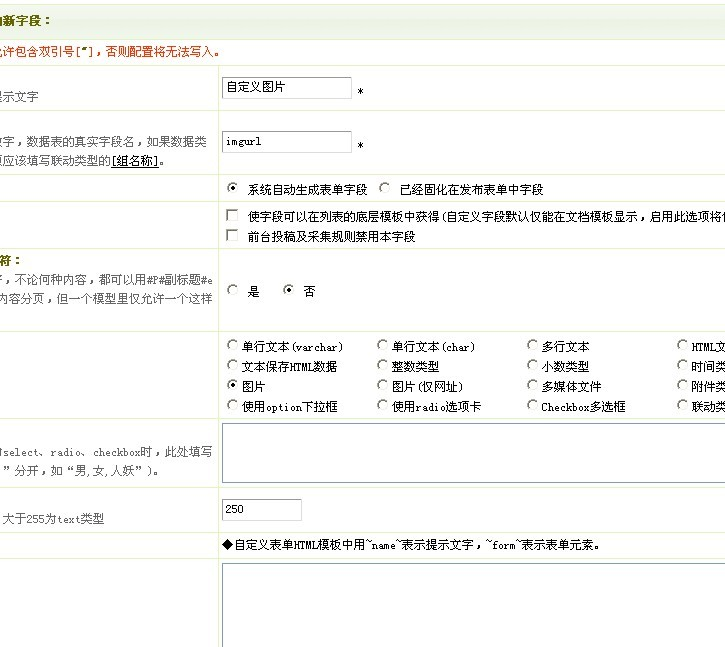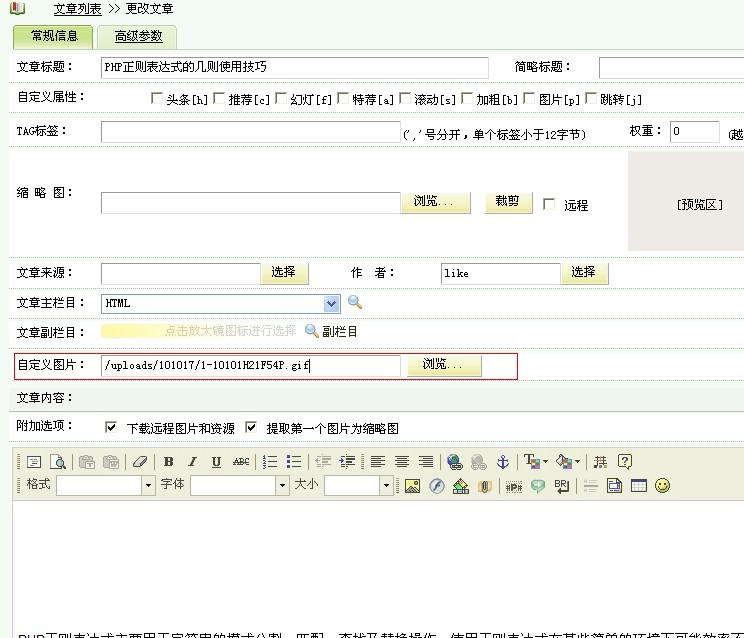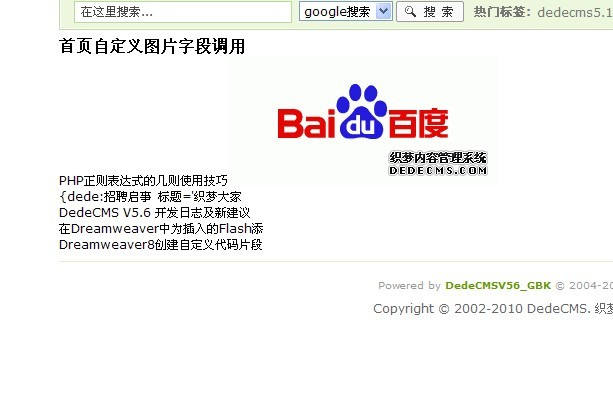# DedeCMS织梦自定义图片字段调用出现{dede:img ..}

{dede:img text='' width='270' height='129'} /uploads/101017/1-10101H21F54P.gif {/dede:img} 这样的格式！

1）创建自定义函数

function GetOneImgUrl($img,$ftype=1){
if($img <> ''){$dtp = new DedeTagParse();
$dtp->LoadSource($img);
if(is_array($dtp->CTags)){ foreach($dtp->CTags as $ctag){ if($ctag->GetName()=='img'){
$width =$ctag->GetAtt('width');
$height =$ctag->GetAtt('height');
$imgurl = trim($ctag->GetInnerText());
$img = ''; if($imgurl != ''){
if($ftype==1){$img .= $imgurl; } else{$img .= '<img src="'.$imgurl.'" width="'.$width.'" height="'.$height.'" />'; } } } } }$dtp->Clear();
return $img; } }  保存后关闭即可。 2）函数调用方法 问：我们创建好函数以后，那么这个函数要如何来调用呢？ 答：本函数的调用方法为： [field:自定义图片字段名 function="GetOneImgUrl(@me,显示类型)"/] 比如：我们的自定义图片字段名为 imgurl ，那么调用方式有以下两种： [field:imgurl function='GetOneImgUrl(@me,0)'/] <!--调用图片的信息，自动调用宽度和高度（已包含Html代码的<img />标签）--> [field:imgurl function='GetOneImgUrl(@me,1)'/] <!--只调用图片地址，一般可用于背景图嵌套--> 二、示例演示 1）模型字段添加 进入网站后台，点击[核心]——[内容模型管理]，点击“普通文章”的“更改”按钮，进入编辑界面，切换到“字段管理”选项，点击“添加新字段”，如图：创建好字段以后，我们在增加或编辑文档时候，那么就会有这个字段了，如图：上传好你要显示的图片以后，保存即可。 2）前台调用 原本我们前台调用这个字段的方法，就是通过arclist标签的 channelid 与 addfields 两个参数来配合调用（点击查看arclist的标签详解），这里不再重复的一一熬述。但大体的自定义字段的方法是这样的： {dede:arclist row='2' channeld='1' addfields='自定义字段1,自定义字段2'} 底层模板 insertext {/dede:arclist} 运用到我们刚才的这个函数，调用方法为： {dede:arclist row='5' orderby='id' channelid='1' addfields='imgurl'} <li>[field:title/][field:imgurl function='GetOneImgUrl(@me,0)'/]</li> {/dede:arclist}  或者使用sql语句查询： {dede:arclist typeid='~id~' flag = 'p'} [field:id runphp="yes"]$row = $GLOBALS['dsql']->GetOne("Select img From dede_addonimages where aid=@me"); @me =$row['img'];
@me=GetOneImgUrl(@me,1);
[/field:id]
{/dede:arclist}